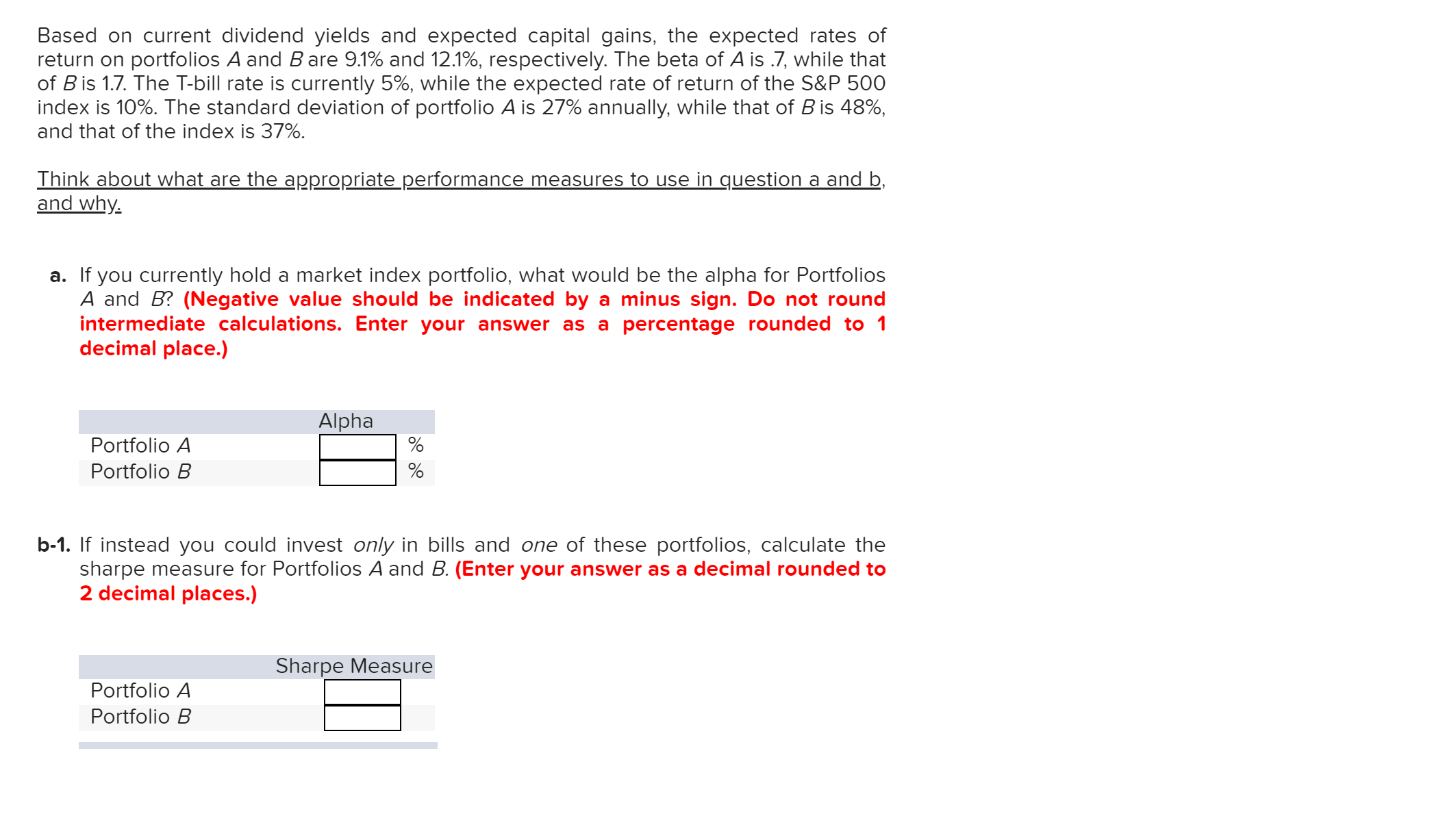# Based on current dividend yields and expected capital gains, the expected rates ofreturn on portfolios A and B are 9.1% and 12.1%, respectively. The beta of A is .7, while thatof B is 1.7. The T-bill rate is currently 5%, while the expected rate of return of the S&P 500index is 10%. The standard deviation of portfolio A is 27% annually, while that of B is 48%,and that of the index is 37%Think about what are the appropriate performance measures to use in question a and band whya. If you currently hold a market index portfolio, what would be the alpha for PortfoliosA and B? (Negative value should be indicated by a minus sign. Do not roundintermediate calculations. Enter your answer as a percentage rounded to 1decimal place.)AlphaPortfolio APortfolio Bb-1. If instead you could invest only in bills and one of these portfolios, calculate thesharpe measure for Portfolios A and B. (Enter your answer as a decimal rounded to2 decimal places.)Sharpe MeasurePortfolio APortfolio B

Question
10 views

need helphelp_outlineImage TranscriptioncloseBased on current dividend yields and expected capital gains, the expected rates of return on portfolios A and B are 9.1% and 12.1%, respectively. The beta of A is .7, while that of B is 1.7. The T-bill rate is currently 5%, while the expected rate of return of the S&P 500 index is 10%. The standard deviation of portfolio A is 27% annually, while that of B is 48%, and that of the index is 37% Think about what are the appropriate performance measures to use in question a and b and why a. If you currently hold a market index portfolio, what would be the alpha for Portfolios A and B? (Negative value should be indicated by a minus sign. Do not round intermediate calculations. Enter your answer as a percentage rounded to 1 decimal place.) Alpha Portfolio A Portfolio B b-1. If instead you could invest only in bills and one of these portfolios, calculate the sharpe measure for Portfolios A and B. (Enter your answer as a decimal rounded to 2 decimal places.) Sharpe Measure Portfolio A Portfolio B fullscreen
check_circle

Step 1

A.

Calculate the alpha of portfolios as follows:

Portfolio A:

Step 2

Portfolio B:

Step 3

B.

Calculate the reward to volatility rati...

### Want to see the full answer?

See Solution

#### Want to see this answer and more?

Solutions are written by subject experts who are available 24/7. Questions are typically answered within 1 hour.*

See Solution
*Response times may vary by subject and question.
Tagged in